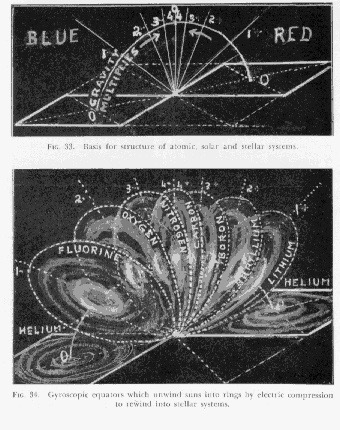# Universal Direction - page 150

It is well to recall the fact that the concept of motion is universally simultaneous and its expression sequential.

The attraction of gravitation and the repulsion of radiation are instantaneous and continuous. Time is not consumed in transferring the concept of any intended expression of energy throughout the dimensionless universe.

The action which is an expression of the intent has dimension and therefore consumes time.

In other words, the concept image of all idea of thinking is universally simultaneous, but the production and reproduction of the form of idea, through motion, is a series of events which are sequential.

All opposite effects are positive and negative, or more and less, or plus and minus. [See syntropy and entropy]

Like all other effects of motion in the universe of dimension, direction has its opposites.

In this universe of more and less, the opposites of that effect of motion which we call "direction" are north and south.

All direction begins at the pressureless plane of south and ends at the opposed pressures plane of north.

North is the electro-positive plus direction of the more, and south is the electro-negative minus direction of the less.

The plus electric force is always away from the south toward north and the minus magnetic force is always away from the north and back to the south.

North begins at south at the point where energy expresses itself by impact against the inertial plane.

At this point, energy simultaneously measures the intensity of its concept in harmonic circles on inertial planes over areas which become the bases of opposing cones of energy. It also measures the intensity of its concept at the intersection of the primary and secondary vertical inertial planes at altitudes which become the apices of those opposing cones. The axes of those cones then become the charging pole areas of all mass and the line of its forming wave. [See Universal Direction - page 155 for illustration of cube parts. See Universal Direction - page 151 for illustration of cones within cubes.]

Energy simultaneously measures its volume intensity by connecting the circular inertial bases of conceptual cones with their apices. [See 5.7 - Generation of Mass for illustration of harmonic circles and 'circular inertial bases']

The harmonic circles which measure the bases of cones are the octave conceptual dimensions of the energy wave of measurable dimension.

The apices of the cones are the overtones of the wave.

The line of increasing force is from the magnetic base following the northerly electric stream to the apex of every cone of energy. [See syntropy]

Conversely, the line of decreasing force is from the electric apex following the southerly magnetic stream to the base of every cone of energy. [See entropy]

South is the direction toward stability and the force of non-motion of substance in inertia.

South is the plane of inertia where every expression of energy begins to gather its force from the expanded area of its harmonic circles, with the intent of overcoming the inertia of substance by concentrating it into harmonic spheres at its overtone points of north.

All energy expresses itself in spiral waves. [See Figure 3.18 - All Motion is Vortex Motion]

The inertial plane of every wave is its south.

Every direction away from the inertial plane is north.

South is the plane of inertia which apparently divides, as inertia is overcome, into two series of opposing planes of motion, the positive and the negative series. [See Polarization]

Each series of opposing planes of motion tilt spirally away from inertia on opposite sides until the harmonic circles which define their areas, have not only attained angles of 90° to it, but have turned half way around. This process can best be visualized by placing two discs together and viewing them edgewise as lines. Then simultaneously tilt and rotate these discs away from each other on opposite edges until they are seen as two circular planes.

150